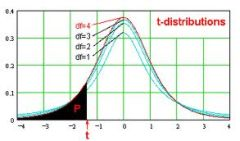# Effect Size Calculator – Cohen’s d

Instructions: This Effect Size Calculator will compute the value of Cohen's d for you, showing all the steps. Please provide the information required below:Sample Mean ($$\bar X$$)Population Mean ($$\mu$$)Population St. Dev. ($$\sigma$$)

Cohen's d corresponds to a widely used measure of effect size , that is used as an alternative (or complement) to the processes of hypothesis testing and calculation of p-values. Hypothesis testing has been subject to criticism because the p-values that are used to estimate the significance of an effect is heavily depending upon the sample size $$n$$.

### How do you calculate the effect size?

A effect size measure attempts to assess the size of the effect in a way that is not influenced heavily by the sample size. Cohen's d is computed by using the following formula:

$d = \frac{|\bar X - \mu|}{\sigma}$

Cohen's D is typically used for t-tests, where the response variable is a scale variable (measured at the ratio level or the interval level), although it can be also used for z-tests. If you know the value of the t-statistic and you know the number of degrees of freedom, you can compute this effect size measure .

### In practice, what is a good effect size?

There are no good or bad effect sizes. The effect size indicates the strength of the association between the independent and dependent variable. The higher the effect size, the stronger is the association.

The are conventions to assess the size of an effect. Cohen's conventions are that a effect size d around 0.2 indicates a small effect size, an effect size d around 0.5 indicates a medium effect size, and an effect size d of around 0.8 indicates a large effect size.### Other effect size measures

There are other measures of effect size for the case of dependent variables that are nominal or ordinal, such as the the Phi Coefficient , the Lambda coefficient , Cramer's V coefficient and the Gamma coefficient .